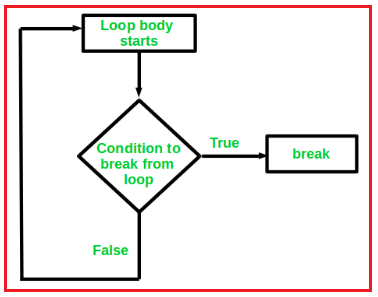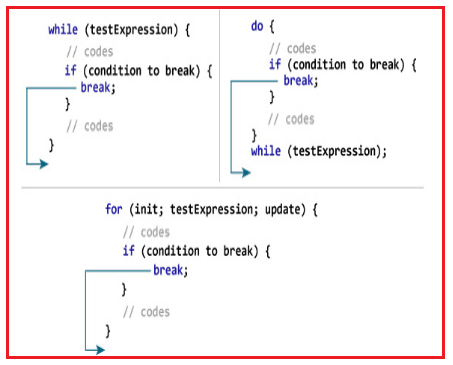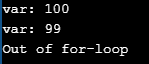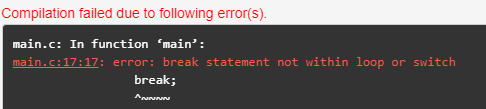# Break Statement in C Language

## Break Statement in C Language with Examples

In this article, I am going to discuss the Break Statement in C Language with Examples. Please read our previous articles, where we discussed For Loop in C Language with Examples. Before understanding the Break statement, let us first understand what are jump statements, its type in C.

##### What are Jump Statements in C Language?

Jump statements are used to modify the behavior of conditional and iterative statements. Jump statements allow you to exit a loop, start the next iteration of a loop, or explicitly transfer program control to a specified location in your program. C provides the following loop for jump statements:

• break
• continue
• return
##### Break Statement in C Language:

The break is a keyword. By using break we can terminate the loop body or switch body. Using break is always optional but it should be placed within the loop body and switch body only. In an implementation where we know the maximum number of repetition but some condition is there where we need to terminate the loop body then we need to go for a break.

The break statement provides a convenient way to immediately exit from a loop (FOR, WHILE, REPEAT), CASE, or SWITCH statement without resorting to the GOTO statement. The break statement ends the loop immediately when it is encountered. The break statement is almost always used with the if…else statement inside the loop.##### How does the break statement work in C language?Syntax: break;

##### Program to Understand Break Statement in C Language:
```#include <stdio.h>
int main()
{
int var;
for (var =100; var>=10; var --)
{
printf("var: %d\n", var);
if (var==99)
{
break;
}
}
printf("Out of for-loop");
return 0;
}```
###### Output:##### Some tricky questions related to C Break Statement:
###### Question1: What will be the output in the below program?
```#include <stdio.h>
int main()
{
int a = 1;
while(a <= 10)
{
printf("%d ", a);
if(a > 3)
break;
a++;
}
return 0;
}```

Output: 1 2 3 4

This is because whenever the value of a become 4 then the condition becomes true then the break statement will be executed. Whenever the break statement is executed automatically control will pass outside of the loop body.

###### Question2: What will be the output in the below program?
```#include <stdio.h>
int main()
{
int a = 2;
while(a <= 20)
{
printf("%d ", a);
a += 2;
if(a >= 8)
break;
}
return 0;
}```

Output: 2 4 6

###### Question3: What will be the output in the below program?
```#include <stdio.h>
int main()
{
int a = 15;
while(a >= 3)
{
a -= 2;
printf("%d ", a);
if(a <= 9)
break;
}
return 0;
}```

Output: 13 11 9

###### Question4: What will be the output in the below program?
```#include <stdio.h>
int main()
{
int a = 15;
while(a >= 5)
{
printf("%d", a);
if(a <= 10);
break;
a -= 2;
}
return 0;
}```

Output: 15

Note: When the semicolon (;) is available at the end of the line then it become dummy condition that the break statement is placing directly outside the condition in the loop.

###### Question5: What will be the output in the below program?
```#include <stdio.h>
int main()
{
int a = 8;
while(a <= 80);
{
printf("%d", a);
if(a >= 20);
break;
a += 2;
}
return 0;
}```
###### Output:Note: When the semicolon is available at the end of the while then it becomes a dummy loop. When the dummy loop is created then the compiler will create a new body without any statements and the current body becomes outside so automatically break become outside and as we know we cannot use break outside of the loop body.